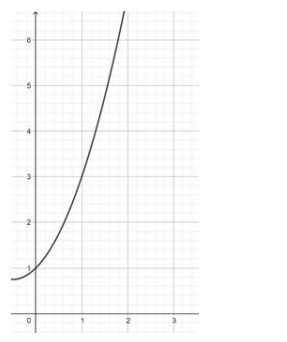# Prove that the functionQuestion:

Prove that the function $f: N \rightarrow N: f(n)=\left(n^{2}+n+1\right)$ is one - one but not onto.

Solution:

In the given range of $N f(x)$ is monotonically increasing.

$\therefore f(n)=n^{2}+n+1$ is one one.But Range of $f(n)=[0.75, \infty) \neq N$ (codomain)

Hence, $f(n)$ is not onto.

Hence, proved that the function $f: N \rightarrow N: f(n)=\left(n^{2}+n+1\right)$ is one - one but not onto.